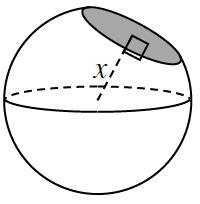### Home > CCG > Chapter 11 > Lesson 11.2.2 > Problem11-96

11-96.While making his lunch, Alexander sliced off a portion of his grapefruit. If the area of the cross-section of the slice (shaded at right) was $3\text{ in.}^2$, and if the diameter of the grapefruit was $5$ inches long, find the distance between the center of the grapefruit and the slice. Assume the grapefruit is a sphere.

$\text{Area of slice}=\pi r^2$

$3 = πr^2$

$\frac{ 3 }{\pi }=r^2$

$\sqrt{\frac{3}{\pi}}=r=\text{radius of slice}$

If the $\text{diameter of sphere}=5$ inches, then the $\text{radius}=x=2.5$ inches.

By the Pythagorean Theorem $x^2+\left(\sqrt{\frac{3}{\pi}}^2\right)=2.5^2$

$x^2+\frac{ 3 }{\pi }=6.25$

$x = 2.3$ in